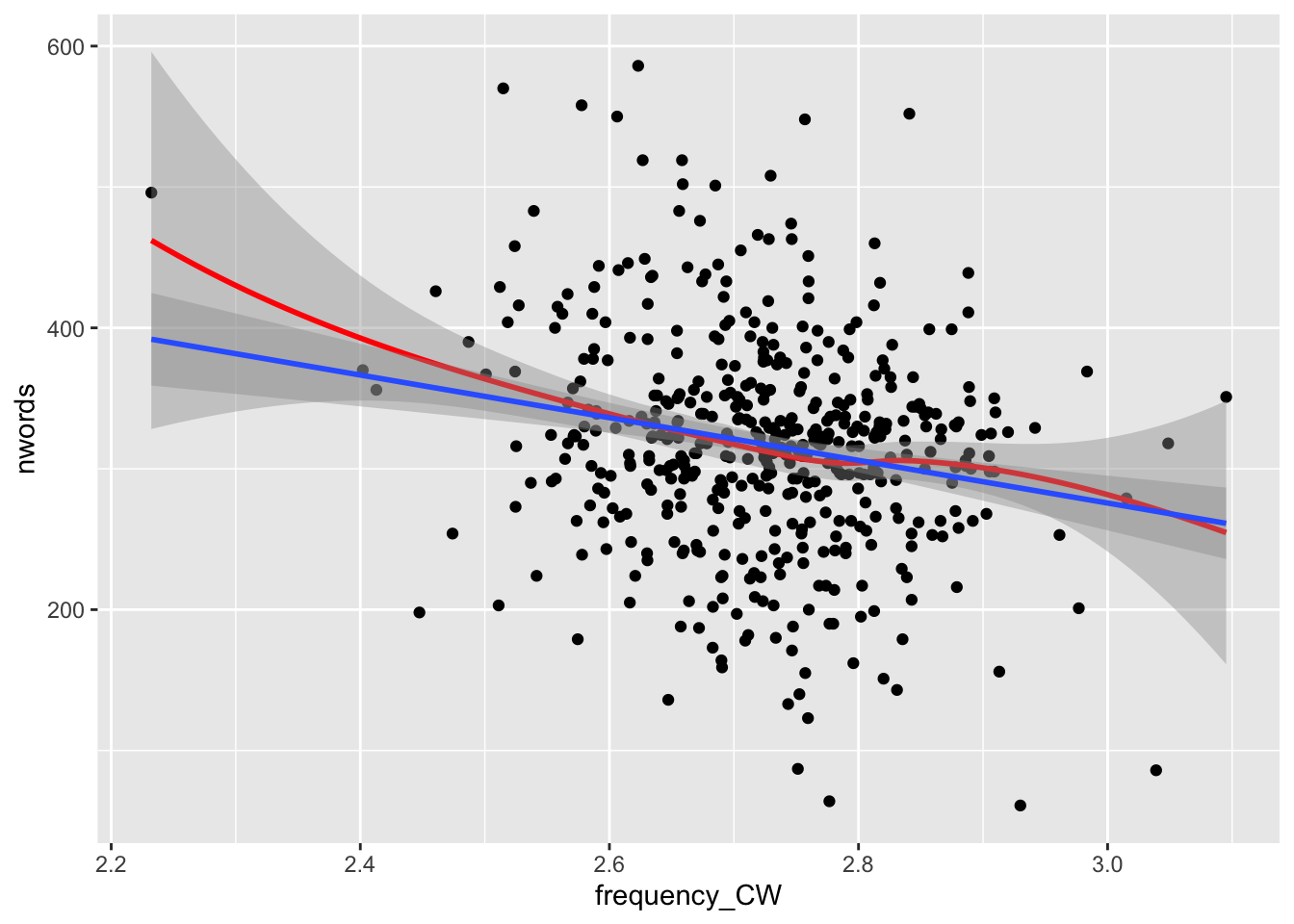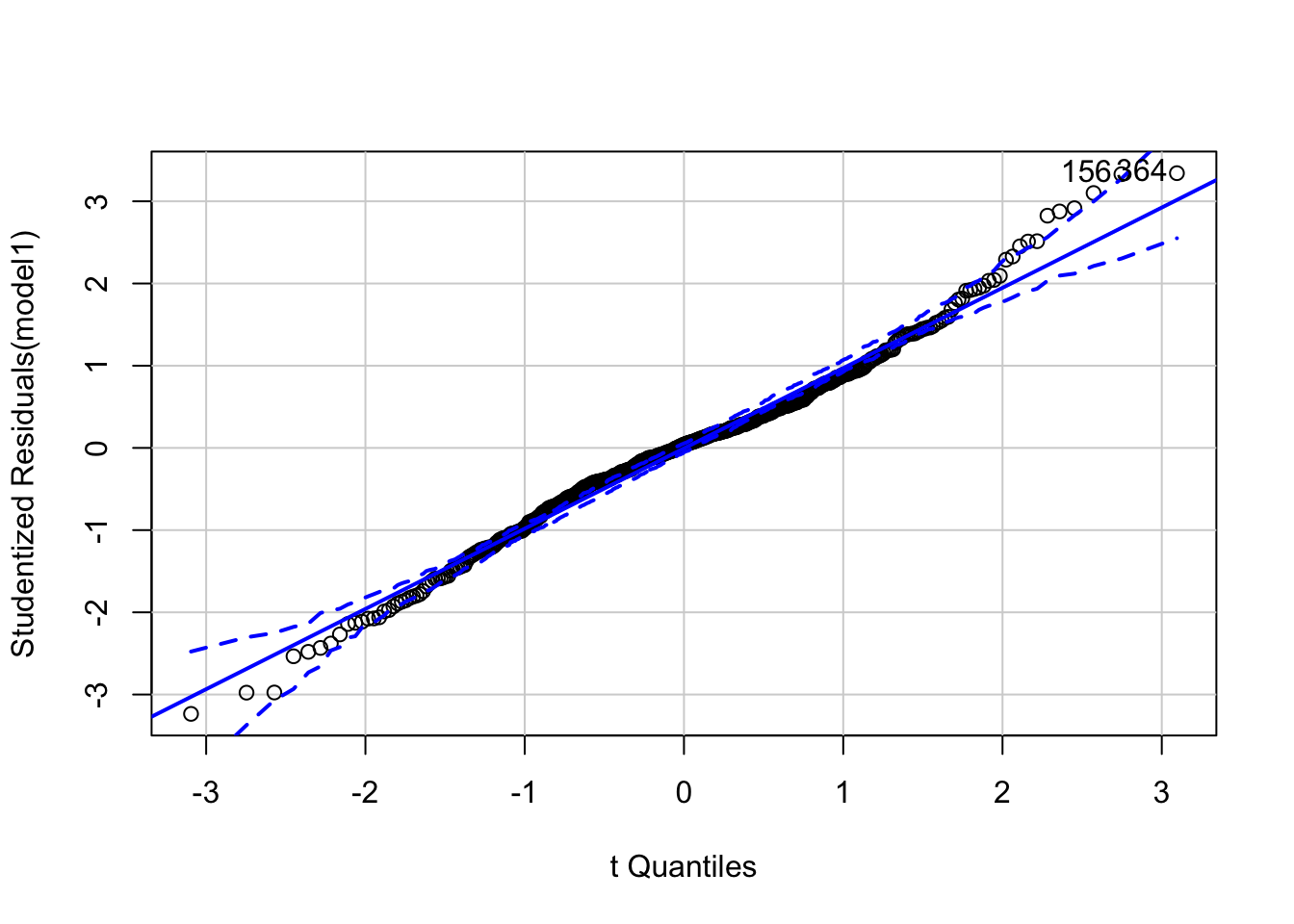## Regression: Predicting dependent variable values based on independent variable values

To fully understand the information on this page, be sure to read the previous tutorial on correlation.

Regression is used for value prediction. For example, lets imagine that we have a set of writing proficiency scores (dependent variable) and corresponding mean word frequency values (which are a measure of lexical sophistication; this would be an independent variable). Regression allows us to predict the writing proficiency scores based on the mean frequency values. The stronger the relationship is between the two values, the better the predictions will be.

Conceptually (and mathematically), regression is related to correlation. The main difference is that the results of a regression include more information, namely the characteristics of the line of best fit through the data.

In this tutorial, we are going to use average word frequency scores (frequency_CW) to predict scores for nwords (which we are using as a [flawed] proxy for proficiency) in a corpus of learner argumentative essays.

### Assumptions

The assumptions for (single) linear regression are almost the same as for correlations.

The main assumptions of (single) linear regression are:

• The variables must be continuous (see other tests for ordinal or categorical data)

• The variables must have a linear relationship

• There are no outliers (or there are only minimal outliers in large samples)

• The residuals (i.e., the prediction errors) are normally distributed

### Checking assumptions

First, we will load our data:

``````library(ggplot2) #load ggplot2
summary(cor_data) #get descriptive statistics for the dataset``````
``````##      Score       pass.fail  Prompt       nwords       frequency_AW
##  Min.   :1.000   fail:223   p1:240   Min.   : 61.0   Min.   :2.963
##  1st Qu.:3.000   pass:257   p2:240   1st Qu.:273.0   1st Qu.:3.187
##  Median :3.500                       Median :321.0   Median :3.237
##  Mean   :3.427                       Mean   :317.7   Mean   :3.234
##  3rd Qu.:4.000                       3rd Qu.:355.2   3rd Qu.:3.284
##  Max.   :5.000                       Max.   :586.0   Max.   :3.489
##   frequency_CW    frequency_FW   bigram_frequency
##  Min.   :2.232   Min.   :3.598   Min.   :1.240
##  1st Qu.:2.656   1st Qu.:3.827   1st Qu.:1.440
##  Median :2.726   Median :3.903   Median :1.500
##  Mean   :2.723   Mean   :3.902   Mean   :1.500
##  3rd Qu.:2.789   3rd Qu.:3.975   3rd Qu.:1.559
##  Max.   :3.095   Max.   :4.235   Max.   :1.755``````

Our variables of interest (frequency_CW) and number of words (nwords) are both continuous variables, so we meet the first assumption.

Next, we will test the assumption of linearity using a Loess line (in red) and a line of best fit (in blue). These indicate that our data are roughly linear (because the red line mirrors the blue one). Note that this is the same data from the correlation tutorial, but with the x and y axes flipped.

``````ggplot(cor_data, aes(x = frequency_CW , y=nwords )) +
geom_point() +
geom_smooth(method = "loess",color = "red") + #this is a line of best fit based on a moving average
geom_smooth(method = "lm") #this is a line of best fit based on the enture dataset``````Looking again at the scatterplot, we see that there are a few outliers. However, given the size of our dataset, these shouldn’t be a problem.

We will check the distribution of the residuals AFTER running the regression (we have to run the regression to get the residuals).

### Conducting a linear regression

Linear regression is very easy to do in R. We simply use the lm() function:

``````#define model1 as a regression model using frequency_CW to predict nwords
model1 <- lm(nwords ~ frequency_CW, data = cor_data)``````

We then can see the model summary using the summary() function:

``````#define model1 as a regression model using frequency_CW to predict nwords
summary(model1)``````
``````##
## Call:
## lm(formula = nwords ~ frequency_CW, data = cor_data)
##
## Residuals:
##      Min       1Q   Median       3Q      Max
## -245.502  -42.924    3.102   40.703  253.254
##
## Coefficients:
##              Estimate Std. Error t value Pr(>|t|)
## (Intercept)    729.93      90.92   8.028 7.75e-15 ***
## frequency_CW  -151.42      33.37  -4.538 7.20e-06 ***
## ---
## Signif. codes:  0 '***' 0.001 '**' 0.01 '*' 0.05 '.' 0.1 ' ' 1
##
## Residual standard error: 76.73 on 478 degrees of freedom
## Multiple R-squared:  0.0413, Adjusted R-squared:  0.03929
## F-statistic: 20.59 on 1 and 478 DF,  p-value: 7.201e-06``````

There is a wealth of information provided by this output. For the purposes of this tutorial, we are interested in five particular pieces, including the Estimate(s), the Std. Error, the p-value, the Multiple R-squared, and the Adjusted R-squared.

#### Estimate(s)

The estimate(s) provide the information needed to construct the regression line (and therefore predict nwords values based on frequency_CW values).

The intercept estimate value (in this case, 729.93) indicates the value for nwords when frequency_CW = 0 (this is the y - intercept).

The frequency_CW estimate indicates the slope of the line of best fit. For every decrease of 151.41 in nwords, frequency_CW values will increase by 1.

For any frequency_CW value, we can predict the nwords value using the following formula: nwords = (frequency_CW value * -151.42) + 729.93. So, if our frequency value is 2.8, we will predict that nwords will be 305.954 (see below for this calculation)

``(2.8*-151.42) + 729.93``
``##  305.954``

#### Standard Error (Std. Error)

The standard error figures indicate the standard deviation in the predicted values. Higher standard error indicates a less optimal model. The standard error for the intercept is 90.92, and the standard error for frequency_CW is 33.37.

#### Probability value (p-value)

This indicates the likelihood of observing this data if there were no relationship between our two variables. In this case, the p=value is quite low (7.201e-06, or .000007201, or .0007201%).

#### Multiple R-squared

This is the effect size for regressions. It is the squared value of the correlation coefficient, and indicates the amount of shared variance between the two variables. In this case, frequency_CW explains 4.13% of the variance in nwords scores (R-squared = .0413), which is quite small.

This is the effect size adjusted for the number of predictor variables used. As we increase variables, the adjusted R-squared will be progressively lower than the unadjusted value. In this case, our Adjusted R-squared value is .03929.

### Checking the assumption of normality (of residuals)

Now that we have run the model, we can check the assumption of normality (of the residuals) using a qq plot. A perfectly normal distribution of residuals would be when all data points fall perfectly on the line. As we can see, we have some outliers (particularly on the right side of the graph), but the distribution is roughly normal.

``````library(car)
qqPlot(model1)````````##  156 364``